# Count total number of Palindromic Subsequences

Count the total number of Palindromic Subsequences: Here, we are going to learn how to find the total number of Palindromic sub-sequences in a string? This is a standard interview problem that can be featured in any interview coding rounds.
Submitted by Radib Kar, on June 14, 2020

Problem statement:

Given a string str, find total number of possible palindromic sub-sequences. A sub-sequence does not need to be consecutive, but for any xixj i<j must be valid in the parent string too. Like "icl" is a subsequence of "includehelp" while "ple" is not.

Input:

The first line of input contains an integer T, denoting the no of test cases then T test cases follow. Each test case contains a string str.

Output:

For each test case output will be an integer denoting the total count of palindromic subsequence which could be formed from the string str.

Constraints:

```1 <= T <= 100
1 <= length of string str <= 300
```

Example:

```Input:
Test case: 2

First test case:
Input string:
"aaaa"

Output:
Total count of palindromic subsequences is: 15

Second test case:
Input string:
"abaaba"

Output:
Total count of palindromic subsequences is: 31
```

Explanation:

Test case 1:

Input: "aaaa"

The valid palindromic subsequences are shown below,

Marked cells are character taken in subsequence:

Count=1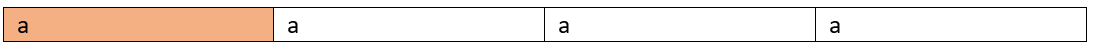Count=2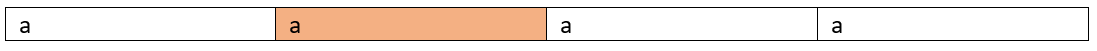Count=3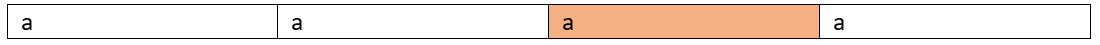Count=4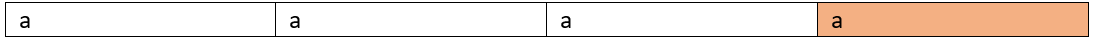Count=5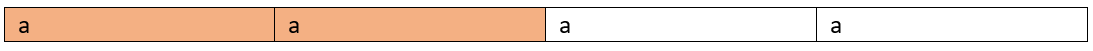Count=6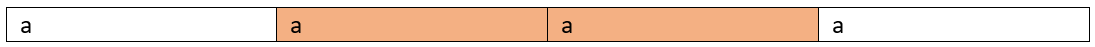Count=7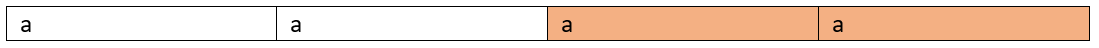Count=8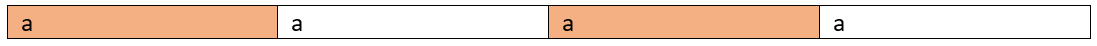Count=9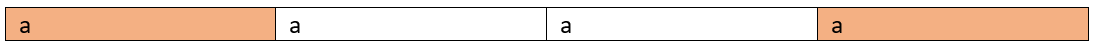Count=10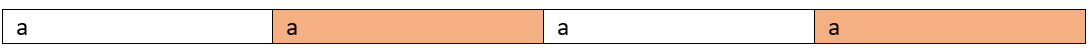Count=11

So on...
Total 15 palindromic sub-sequences
Actually in this case since all the character is same each and every subsequence is palindrome here.
For the second test case
Few sub-sequences can be
"a"
"b"
"a"
"aba"
So on
Total 31 such palindromic subsequences

Solution approach

This can be solved by using DP bottom up approach,

1. Initialize dp[n][n] where n be the string length to 0
2. Fill up the base case, Base case is that each single character is a palindrome itself. And for length of two, i.e, if adjacent characters are found to be equal then dp[i][i+1]=3, else if characters are different then dp[i][i+1]=2
To understand this lets think of a string like "acaa"
Here dp=2 because there's only two palindrome possible because of "a" and "c".
Whereas for dp value will be 3 as possible subsequences are "a", "a", "aa".
```for i=0 to n
// for single length characters
dp[i][i]=1;
if(i==n-1)
break;
if(s[i]==s[i+1])
dp[i][i+1]=3;
else
dp[i][i+1]=2;
end for
```
3. Compute for higher lengths,
```for len=3 to n
for start=0 to n-len
int end=start+len-1;
// start and end is matching
if(s[end]==s[start])
// 1+subsequence from semaining part
dp[start][end]=1+dp[start+1][end]+dp[start][end-1];
else
dp[start][end]=dp[start+1][end]+dp[start][end-1]-dp[start+1][end-1];
end if
end for
end for
```
4. Final result is stored in dp[n-1];

So for higher lengths if starting and ending index is the same then we recur for the remaining characters, since we have the sub-problem result stored so we computed that. In case start and end index character are different then we have added dp[start+1][end] and dp[start][end-1] that's similar to recur for leaving starting index and recur for leaving end index. But it would compute dp[start+1][end-1] twice and that why we have deducted that.

For proper understanding you can compute the table by hand for the string "aaaa" to understand how it's working.

C++ Implementation:

```#include <bits/stdc++.h>
using namespace std;

int countPS(string s)
{

int n = s.length();
int dp[n][n];

memset(dp, 0, sizeof(dp));

for (int i = 0; i < n; i++) {
dp[i][i] = 1;
if (i == n - 1)
break;

if (s[i] == s[i + 1])
dp[i][i + 1] = 3;
else
dp[i][i + 1] = 2;
}

for (int len = 3; len <= n; len++) {
for (int start = 0; start <= n - len; start++) {
int end = start + len - 1;
if (s[end] == s[start]) {
dp[start][end] = 1 + dp[start + 1][end] + dp[start][end - 1];
}
else {
dp[start][end] = dp[start + 1][end] + dp[start][end - 1] - dp[start + 1][end - 1];
}
}
}

return dp[n - 1];
}

int main()
{
int t;
cout << "Enter number of testcases\n";
cin >> t;

while (t--) {
string str;
cout << "Enter the input string\n";
cin >> str;

cout << "Total Number of palindromic Subsequences are: " << countPS(str) << endl;
}

return 0;
}
```

Output:

```Enter number of testcases
2
Enter the input string
aaaa
Total Number of palindromic Subsequences are: 15
Enter the input string
abaaba
Total Number of palindromic Subsequences are: 31
```

What's New

Top Interview Coding Problems/Challenges!

Languages: » C » C++ » C++ STL » Java » Data Structure » C#.Net » Android » Kotlin » SQL
Web Technologies: » PHP » Python » JavaScript » CSS » Ajax » Node.js » Web programming/HTML
Solved programs: » C » C++ » DS » Java » C#
Aptitude que. & ans.: » C » C++ » Java » DBMS
Interview que. & ans.: » C » Embedded C » Java » SEO » HR
CS Subjects: » CS Basics » O.S. » Networks » DBMS » Embedded Systems » Cloud Computing
» Machine learning » CS Organizations » Linux » DOS
More: » Articles » Puzzles » News/Updates